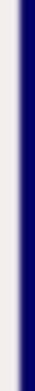homework help forum today's math problem links utilities previous math problem feedback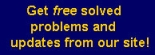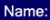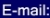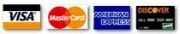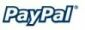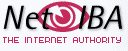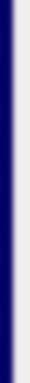# MATH HOMEWORK HELP

The charge for maintaining a checking account at a certain bank is \$12 per month plus 8 cents per check written. A competing bank charges \$6 per month plus 20 cents per check. For how many checks per month are the total charges the same at the two banks.

Solution: Let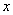be the number of checks per month. Then, we need to solveMGT - Your source of Math and Statistics Homework Help